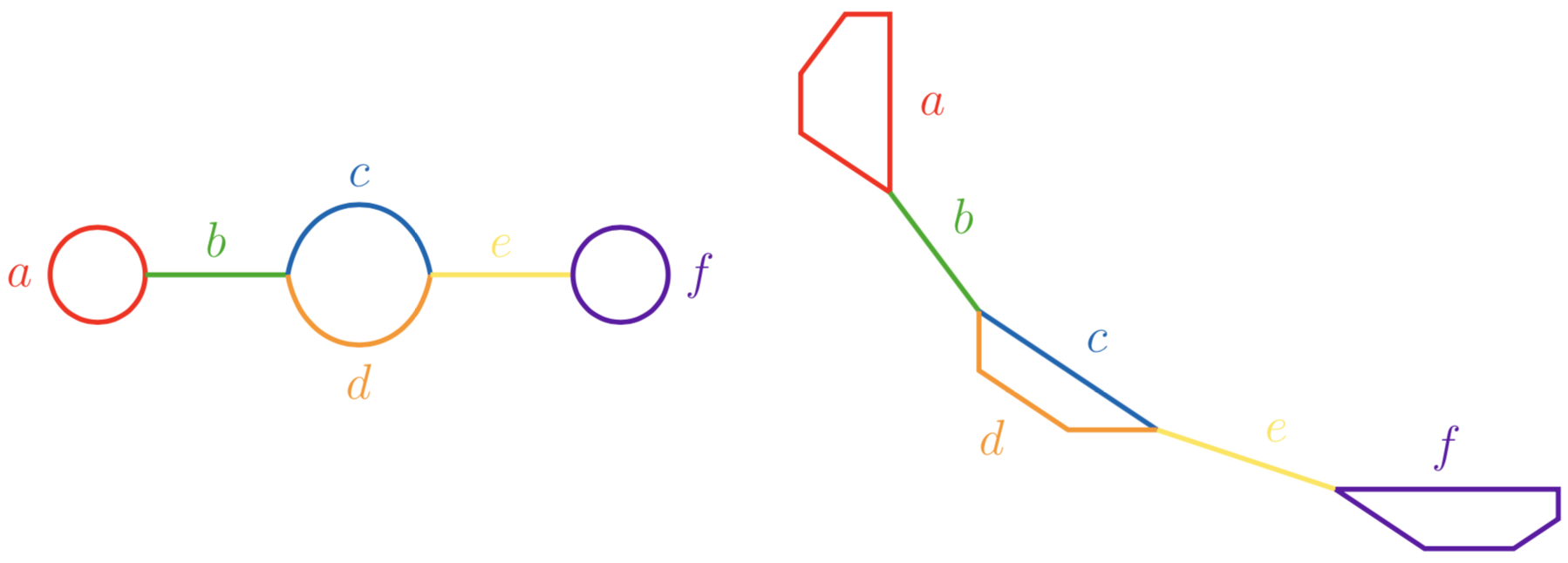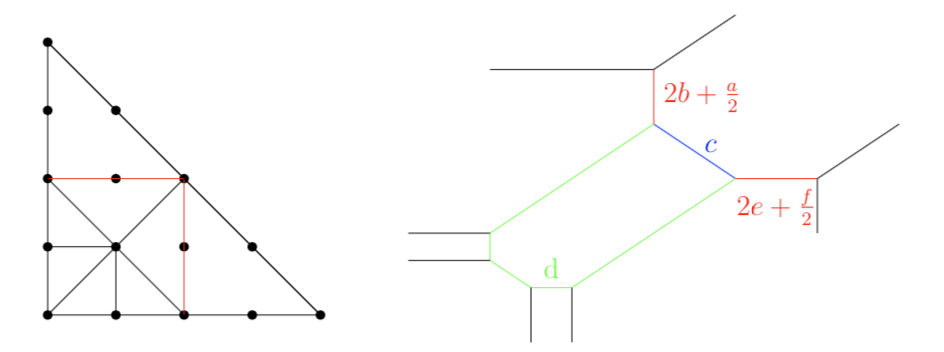# Type (212)¶

## Plane quartics of type (212)¶

A tropical curve of type (212) is of the formDue to symmetry, we assume $$d\geq c$$. It is embeddable as a tropical quartic curve in $$\mathbb{R}^2$$ if $$c<d\leq 2c$$. However, curves with $$c=d$$ are realizibly hyperelliptic, which is why we will ignore them in this context.

## Quartics of type (212) with $$d>2c$$¶

To realize curves with $$d>2c$$, we simply consider the degenerate tropical curve given by the quartic polynomial:

$\begin{split}g&= t^{a+6b+2c}\cdot x^4 + t^{6e+f+2c}\cdot y^4 +x^2y^2+ 2\cdot xy^2 + (1-t^{2b}) y^2+ 2\cdot x^2y \\ & \quad +(1-t^{2e})\cdot x^2 + t^{-c} xy+ t^{\frac{-d+4c-1}{2}}y+ t^{\frac{-d+4c-1}{2}} x+ t^{-d+3c},\end{split}$whose red edges reveal two lollis with wanted egdge and cycle lengths after modification $$x\mapsto x+1-t^b$$ and $$y\mapsto y+1-t^e$$.